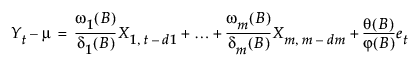Publication date: 05/24/2021

## Statistical Details for Transfer Functions

A typical transfer function model with m inputs can be represented as follows:where

Yt denotes the output series

X1 to Xm denote m input series

et represents the noise series

X1, td1 indicates the series X1 is indexed by t with a d1-step lag

μ represents the mean level of the model

ϕ(B) and θ(B) represent autoregressive and moving average polynomials from an ARIMA model

ωk(B) and δk(B) represent numerator and denominator factors (or polynomials) for individual transfer functions, with k representing an index for the 1 to m individual inputs.

Each polynomial in the above model can contain two parts, either nonseasonal, seasonal, or a product of the two as in seasonal ARIMA. When specifying a model, leave the default 0 for any part that you do not want to include in the model.